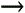Section 13.4. Controlling Recalculation

13.4. Controlling Recalculation

Ordinarily, Excel recalculates a formula whenever you change any of the cells the formula uses, and whenever you open the workbook containing the formula. This behavior is extremely convenient because it ensures that your information never gets out of date. However, it can cause trouble if your worksheet contains time-consuming calculations or extremely large tables of data. In this situation, life can slow to a crawl while Excel recalculates and updates all its formulas.

Tip: You'll know the recalculation is at work when the mouse pointer changes to an hourglass and the word Recalc (followed by the number of cells left to be recalculated) appears on the left side of the formula bar. Even though your cursor looks like an hourglass, you can still enter data and use the menu, but every time you perform any of these activities, it briefly pauses the recalculation process.

With the exponential increase in computer speed (and Microsoft's continuous tweaking of Excel's performance), large worksheets aren't the problem they used to be. However, some Excel aficionados still report problems when crunching data that's thick with formulas. If you're using Excel to plot the path of orbital satellites or to analyze census data, you may be interested in switching off Excel's automatic calculation to make your worksheet more responsive .

To select the calculation mode, choose FormulasCalculationCalculation Options, and then pick an option from the menu.

• Manual turns off all automatic calculation.

• Automatic is the standard way of life for Excel spreadsheets. Excel recalculates formulas whenever a cell changes affects them.

• Automatic Except Data Tables uses automatic recalculation for all formulas except those in data tables (Chapter 14).

In manual recalculation mode, Excel calculates most simple formulas when you enter them. However, if you modify cells that the formula uses, then the program doesn't recalculate the formulainstead, it keeps displaying the old result. When you see the word Calculate in the Status bar, that's your clue that Excel would have ordinarily performed a recalculation, but didn't because of your settings. It means that some data's changed, and a recalculation's a good idea to refresh your formula results.

When you decide you do want to perform your recalculation, you can choose FormulasCalculationCalculate Now (or hit F9) to recalculate all the formulas in the workbook. Alternatively, you can choose FormulasCalculationCalculate Sheet (or the shortcut Shift+F9) to recalculate just those formulas on the current worksheet.

Note: Unfortunately, the recalculation settings are application-wide. When you switch off automatic calculation for one worksheet, it affects any other worksheets you're using. If you don't remember that you've switched off automatic recalculation, you may end up using and printing stale data.Excel 2007[c] The Missing Manual
ISBN: 596527594
EAN: N/A
Year: 2007
Pages: 173